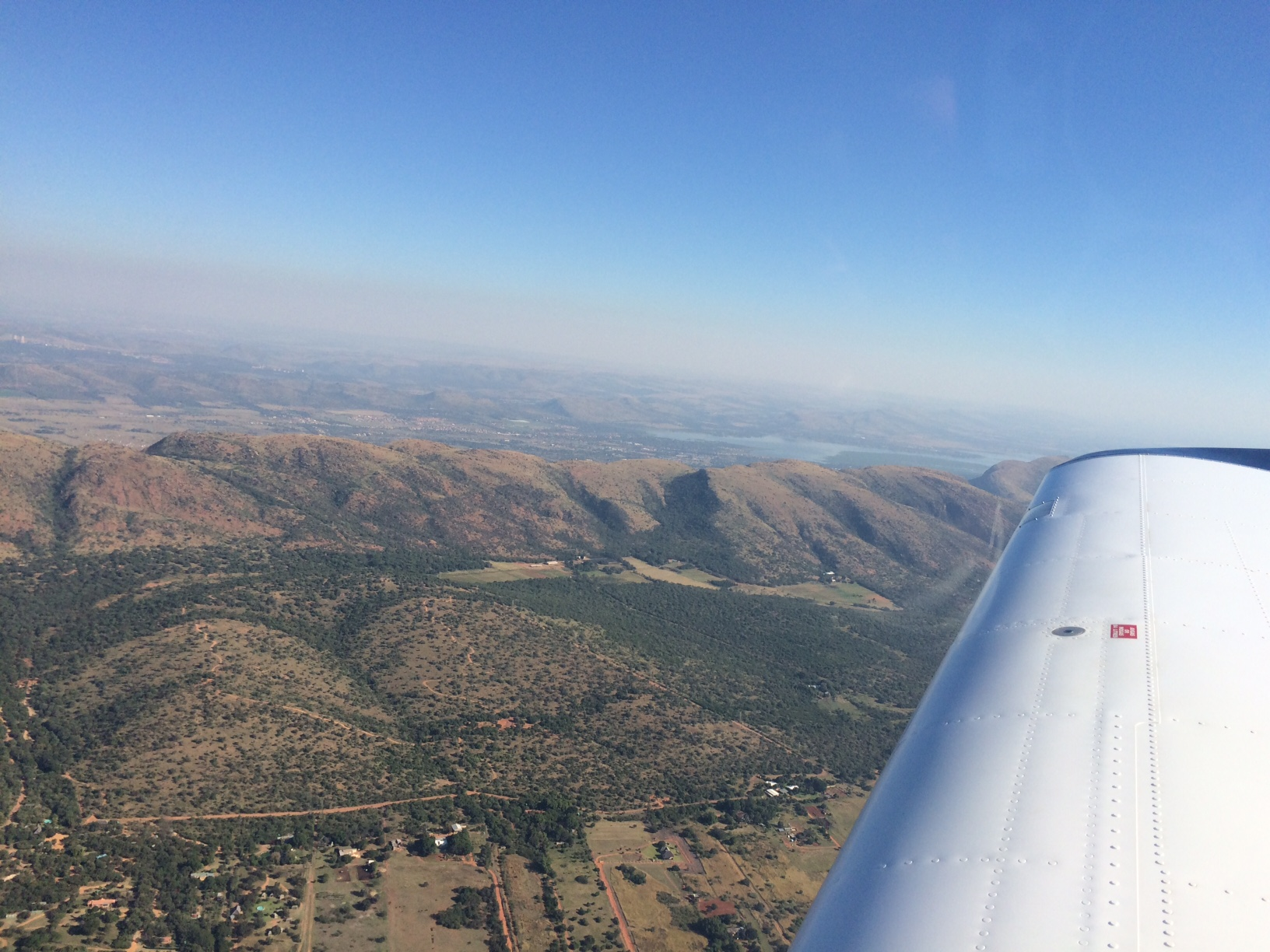Select PageAir pressure is an important player in aviation, as some crucial instruments rely on the measurement of pressure for their operation – most notably the altimeter. In order to obtain accurate altitude readings, and ensure safe flight and obstacle clearance, the altimeter needs to be set, and adjustments need to be made for the actual pressure over a certain area. In aviation, because of the constantly changing atmospheric conditions, the International Standard Atmosphere (ISA) was created to insure safety, in that all aircraft would fly on the same standard settings, even though the actual conditions differ from area to area.

According to the International Standard Atmosphere (ISA), the pressure is set at 1013 hPa. Pressure altitude is the altitude in the standard atmosphere at which the pressure is the same as the existing pressure. In other words, if we have a QNH (barometric pressure at a particular station) of 1023 hPa, then what altitude would this translate to in the standard atmosphere? This equivalent is called pressure altitude

A basic altimeter relies solely on pressure to provide a reading – the diaphragm compresses when the pressure is high (at sea level for example), and releases when the pressure reduces as the aircraft climbs to higher altitudes. When we set the altimeter subscale to 1013 hPa we are flying a flight level (QNE). This setting is used once we have passed the transition altitude, below this we use the local QNH. Unless the QNH is the same as the QNE (1013 hPa), then we must be aware that this difference can produce a significant difference.

Why do we need to know what the pressure altitude is?

Although we use pressure altitude directly when we fly flight levels, it is also used to calculate density altitude, and when using aircraft performance graphs, to determine take off and landing performance.

We can calculate the pressure altitude as follows:

Example data:
Airport elevation: 5500ft
Local QNH: 1023 hPa

Firstly, work out the pressure difference between the ISA standard 1013 hPa and the local QNH:

1023 – 1013 = 10

Multiply by 30 (or whatever the question specifies in an exam e.g. 27) in order to obtain the difference in feet:

10 x 30 = 300ft

Now do we add this or subtract this from the airport elevation?

If the local pressure is higher, then our altimeter thinks we are are at a lower altitude (where pressure is higher), so we need to SUBTRACT the difference from the airport elevation.

If the local pressure is lower, then our altimeter thinks we are are at a higher altitude (where pressure is lower), so we need to ADD the difference from the airport elevation.The column of air at sea level is much greater and heavier, therefore, it exerts more pressure.

The column of air over higher ground is shorter than that over the sea, therefore it is ‘lighter’ and exerts less pressure.